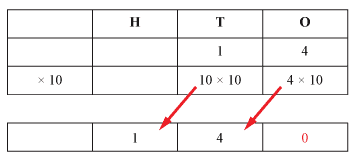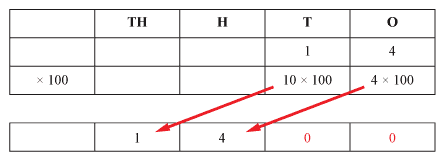## Content description

Multiply and divide decimals by powers of 10 (ACMNA130)

Source: Australian Curriculum, Assessment and Reporting Authority (ACARA)

### Multiply and divide decimals by powers of 10

When a number is multiplied by a power of 10, each digit is multiplied by the same power of 10:

\begin{align*} 25.89 \times 10 &= 20 \times 10 + 5 \times 10 + 0.8 \times 10 + 0.09 \times 10 \\ &= 200 + 50 + 8 + 0.9 \\ &= 258.9\end{align*}

When a number is divided by a power of 10, each digit is divided by the same power of 10:

\begin{align*} 25.89 \div 10 &= 20 \div 10 + 5 \div 10 + \frac{8}{10} \div 10 + \frac{9}{100} \div 10 \\ &= 2 + \frac{5}{10} + \frac{8}{100} + \frac{9}{1000} \\ &= 2.589 \end{align*}

#### Multiplying whole numbers by 10, 100 and 1000

When 14 is multiplied by 10, the 1 in the tens place is multiplied by 10, and the 4 in the ones place is multiplied by 10.

10 × 10 = 100, and 4 × 10 = 40, and we place a zero in the ones place to say that there are 'no ones'.Detailed description

14 × 10 = 140

If we multiply 14 by 100:Detailed description

14 × 100 = 1400
We can extend the pattern:
14 × 10 = 140
14 × 100 = 1400
14 × 1000 = 14 000
14 × 10 000 = 140 000
and so on.